JMSLTM Numerical Library 7.2.0
com.imsl.stat

## Class NonlinearRegression

• ```public class NonlinearRegression
extends Object```
Fits a multivariate nonlinear regression model using least squares. The nonlinear regression model is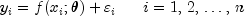where the observed values of the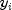constitute the responses or values of the dependent variable, the known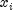are vectors of values of the independent (explanatory) variables,is the vector ofregression parameters, and the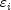are independently distributed normal errors each with mean zero and variance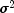. For this model, a least squares estimate ofis also a maximum likelihood estimate of.

The residuals for the model are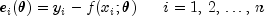A value ofthat minimizes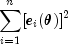is the least-squares estimate ofcalculated by this class. `NonlinearRegression` accepts these residuals one at a time as input from a user-supplied function. This allows `NonlinearRegression` to handle cases whereis so large that data cannot reside in an array but must reside in a secondary storage device.

`NonlinearRegression` is based on MINPACK routines `LMDIF` and `LMDER` by More' et al. (1980). `NonlinearRegression` uses a modified Levenberg-Marquardt method to generate a sequence of approximations to the solution. Let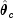be the current estimate of. A new estimate is given by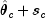where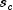is a solution to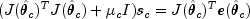Here,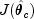is the Jacobian evaluated at.

The algorithm uses a "trust region" approach with a step bound of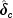. A solution of the equations is first obtained for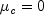. If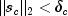, this update is accepted; otherwise,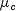is set to a positive value and another solution is obtained. The method is discussed by Levenberg (1944), Marquardt (1963), and Dennis and Schnabel (1983, pages 129 - 147, 218 - 338).

Forward finite differences are used to estimate the Jacobian numerically unless the user supplied function computes the derivatives. In this case the Jacobian is computed analytically via the user-supplied function.

`NonlinearRegression` does not actually store the Jacobian but uses fast Givens transformations to construct an orthogonal reduction of the Jacobian to upper triangular form. The reduction is based on fast Givens transformations (see Golub and Van Loan 1983, pages 156-162, Gentleman 1974). This method has two main advantages: (1) the loss of accuracy resulting from forming the crossproduct matrix used in the equations foris avoided, and (2) the n x p Jacobian need not be stored saving space when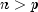.

A weighted least squares fit can also be performed. This is appropriate when the variance of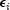in the nonlinear regression model is not constant but instead is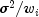. Here,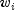are weights input via the user supplied function. For the weighted case, ```NonlinearRegression ``` finds the estimate by minimizing a weighted sum of squares error.

### Programming Notes

Nonlinear regression allows users to specify the model's functional form. This added flexibility can cause unexpected convergence problems for users who are unaware of the limitations of the algorithm. Also, in many cases, there are possible remedies that may not be immediately obvious. The following is a list of possible convergence problems and some remedies. There is not a one-to-one correspondence between the problems and the remedies. Remedies for some problems may also be relevant for the other problems.

1. A local minimum is found. Try a different starting value. Good starting values often can be obtained by fitting simpler models. For example, for a nonlinear function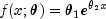good starting values can be obtained from the estimated linear regression coefficients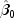and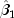from a simple linear regression of ln y on ln x. The starting values for the nonlinear regression in this case would be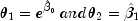If an approximate linear model is unclear, then simplify the model by reducing the number of nonlinear regression parameters. For example, some nonlinear parameters for which good starting values are known could be set to these values. This simplifies the approach to computing starting values for the remaining parameters.
2. The estimate ofis incorrectly returned as the same or very close to the initial estimate.
• The scale of the problem may be orders of magnitude smaller than the assumed default of 1 causing premature stopping. For example, if the sums of squares for error is less than approximately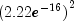, the routine stops. See Example 3, which shows how to shut down some of the stopping criteria that may not be relevant for your particular problem and which also shows how to improve the speed of convergence by the input of the scale of the model parameters.
• The scale of the problem may be orders of magnitude larger than the assumed default causing premature stopping. The information with regard to the input of the scale of the model parameters in Example 3 is also relevant here. In addition, the maximum allowable step size (`setMaxStepsize(double)`) in Example 3 may need to be increased.
• The residuals are input with accuracy much less than machine accuracy causing premature stopping because a local minimum is found. Again see Example 3 to see generally how to change some default tolerances. If you cannot improve the precision of the computations of the residual, you need to use method `setDigits(int)` to indicate the actual number of good digits in the residuals.
3. The model is discontinuous as a function of. There may be a mistake in the user-supplied function. Note that the function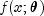can be a discontinuous function of x.
4. The R matrix returned by `getR` is inaccurate. If only a function is supplied try providing the `NonlinearRegression.Derivative`. If the derivative is supplied try providing only `NonlinearRegression.Function`.
5. Overflow occurs during the computations. Make sure the user-supplied functions do not overflow at some value of.
6. The estimate ofis going to infinity. A parameterization of the problem in terms of reciprocals may help.
7. Some components ofare outside known bounds. This can sometimes be handled by making a function that produces artificially large residuals outside of the bounds (even though this introduces a discontinuity in the model function).

Note that the `solve` method must be called prior to calling the "get" member functions, otherwise a `null` is returned.

Example 1, Example 2, Example 3
• ### Nested Class Summary

Nested Classes
Modifier and Type Class and Description
`static interface ` `NonlinearRegression.Derivative`
Public interface for the user supplied function to compute the derivative for `NonlinearRegression`.
`static interface ` `NonlinearRegression.Function`
Public interface for the user supplied function for `NonlinearRegression`.
`static class ` `NonlinearRegression.NegativeFreqException`
A negative frequency was encountered.
`static class ` `NonlinearRegression.NegativeWeightException`
A negative weight was encountered.
`static class ` `NonlinearRegression.TooManyIterationsException`
The number of iterations has exceeded the maximum allowed.
• ### Constructor Summary

Constructors
Constructor and Description
`NonlinearRegression(int nparm)`
Constructs a new nonlinear regression object.
• ### Method Summary

Methods
Modifier and Type Method and Description
`double` `getCoefficient(int i)`
Returns the estimate for a coefficient.
`double[]` `getCoefficients()`
Returns the regression coefficients.
`double` `getDFError()`
Returns the degrees of freedom for error.
`int` `getErrorStatus()`
Gets information about the performance of `NonlinearRegression`.
`double[][]` `getR()`
Returns a copy of the `R` matrix.
`int` `getRank()`
Returns the rank of the matrix.
`double` `getSSE()`
Returns the sums of squares for error.
`void` `setAbsoluteTolerance(double absoluteTolerance)`
Sets the absolute function tolerance.
`void` `setDigits(int nGood)`
Sets the number of good digits in the residuals.
`void` `setFalseConvergenceTolerance(double falseConvergenceTolerance)`
Sets the false convergence tolerance.
`void` `setGradientTolerance(double gradientTolerance)`
`void` `setGuess(double[] thetaGuess)`
Sets the initial guess of the parameter values
`void` `setInitialTrustRegion(double initialTrustRegion)`
Sets the initial trust region radius.
`void` `setMaxIterations(int maxIterations)`
Sets the maximum number of iterations allowed during optimization
`void` `setMaxStepsize(double maxStepsize)`
Sets the maximum allowable stepsize.
`void` `setRelativeTolerance(double relativeTolerance)`
Sets the relative function tolerance
`void` `setScale(double[] scale)`
Sets the scaling array for `theta`.
`void` `setStepTolerance(double stepTolerance)`
Sets the step tolerance used to step between two points.
`double[]` `solve(NonlinearRegression.Function F)`
Solves the least squares problem and returns the regression coefficients.
• ### Methods inherited from class java.lang.Object

`clone, equals, finalize, getClass, hashCode, notify, notifyAll, toString, wait, wait, wait`
• ### Constructor Detail

• #### NonlinearRegression

`public NonlinearRegression(int nparm)`
Constructs a new nonlinear regression object.
Parameters:
`nparm` - An `int` which specifies the number of unknown parameters in the regression.
• ### Method Detail

• #### getCoefficient

`public double getCoefficient(int i)`
Returns the estimate for a coefficient.
Parameters:
`i` - An `int` which specifies the index of a coefficient whose estimate is to be returned.
Returns:
A `double` which contains the estimate for the i-th coefficient or `null` if `solve` has not been called.
• #### getCoefficients

`public double[] getCoefficients()`
Returns the regression coefficients.
Returns:
A `double` array containing the regression coefficients or `null` if `solve` has not been called.
• #### getDFError

`public double getDFError()`
Returns the degrees of freedom for error.
Returns:
A `double` which specifies the degrees of freedom for error or `null` if `solve` has not been called.
• #### getErrorStatus

`public int getErrorStatus()`
Gets information about the performance of `NonlinearRegression`.
Returns:
An `int` specifying information about convergence.

 Value Description 0 All convergence tests were met. 1 Scaled step tolerance was satisfied. The current point may be an approximate local solution, or the algorithm is making very slow progress and is not near a solution, or ```StepTolerance ``` is too big. 2 Scaled actual and predicted reductions in the function are less than or equal to the relative function convergence tolerance ``` RelativeTolerance```. 3 Iterates appear to be converging to a noncritical point. Incorrect gradient information, a discontinuous function, or stopping tolerances being too tight may be the cause. 4 Five consecutive steps with the maximum stepsize have been taken. Either the function is unbounded below, or has a finite asymptote in some direction, or the `maxStepsize` is too small.

`setRelativeTolerance(double)`, `setStepTolerance(double)`, `setMaxStepsize(double)`
• #### getR

`public double[][] getR()`
Returns a copy of the `R` matrix. `R` is the upper triangular matrix containing the `R` matrix from a QR decomposition of the matrix of regressors.
Returns:
A two dimensional `double` array containing a copy of the `R` matrix or `null` if `solve` has not been called.
• #### getRank

`public int getRank()`
Returns the rank of the matrix.
Returns:
An `int` which specifies the rank of the matrix or `null` if `solve` has not been called.
• #### getSSE

`public double getSSE()`
Returns the sums of squares for error.
Returns:
A `double` which contains the sum of squares for error or `null` if `solve` has not been called.
• #### setAbsoluteTolerance

`public void setAbsoluteTolerance(double absoluteTolerance)`
Sets the absolute function tolerance.
Parameters:
`absoluteTolerance` - A `double` scalar value specifying the absolute function tolerance. The tolerance must be greater than or equal to zero. The default value is 4.93e-32.
Throws:
`IllegalArgumentException` - is thrown if `absoluteTolerance` is less than 0
• #### setDigits

`public void setDigits(int nGood)`
Sets the number of good digits in the residuals.
Parameters:
`nGood` - An `int` specifying the number of good digits in the residuals. The number of digits must be greater than zero. The default value is 15.
Throws:
`IllegalArgumentException` - is thrown if `ngood` is less than or equal to 0
• #### setFalseConvergenceTolerance

`public void setFalseConvergenceTolerance(double falseConvergenceTolerance)`
Sets the false convergence tolerance.
Parameters:
`falseConvergenceTolerance` - A `double` scalar value specifying the false convergence tolerance. The tolerance must be greater than or equal to zero. The default value is 2.22e-14.
Throws:
`IllegalArgumentException` - is thrown if `falseConvergenceTolerance` is less than 0

`public void setGradientTolerance(double gradientTolerance)`
Parameters:
`gradientTolerance` - A `double` specifying the gradient tolerance used to compute the gradient. The tolerance must be greater than or equal to zero. The default value is 6.055e-6.
Throws:
`IllegalArgumentException` - is thrown if `gradientTolerance` is less than 0
• #### setGuess

`public void setGuess(double[] thetaGuess)`
Sets the initial guess of the parameter values
Parameters:
`thetaGuess` - A `double` array of initial values for the parameters. The default value is an array of zeroes.
• #### setInitialTrustRegion

`public void setInitialTrustRegion(double initialTrustRegion)`
Sets the initial trust region radius.
Parameters:
`initialTrustRegion` - A `double` scalar value specifying the initial trust region radius. The initial trust radius must be greater than zero. If this member function is not called, a default is set based on the initial scaled Cauchy step.
Throws:
`IllegalArgumentException` - is thrown if `initialTrustRegion` is less than or equal to 0
• #### setMaxIterations

`public void setMaxIterations(int maxIterations)`
Sets the maximum number of iterations allowed during optimization
Parameters:
`maxIterations` - An `int` specifying the maximum number of iterations allowed during optimization. The value must be greater than 0. The default value is 100.
Throws:
`IllegalArgumentException` - is thrown if `maxIterations` is less than or equal to 0
• #### setMaxStepsize

`public void setMaxStepsize(double maxStepsize)`
Sets the maximum allowable stepsize.
Parameters:
`maxStepsize` - A nonnegative `double` value specifying the maximum allowable stepsize. The maximum allowable stepsize must be greater than zero. If this member function is not called, maximum stepsize is set to a default value based on a scaled `theta`.
Throws:
`IllegalArgumentException` - is thrown if `maxStepsize` is less than or equal to 0
• #### setRelativeTolerance

`public void setRelativeTolerance(double relativeTolerance)`
Sets the relative function tolerance
Parameters:
`relativeTolerance` - A `double` scalar value specifying the relative function tolerance. The relative function tolerance must be greater than or equal to zero. The default value is 1.0e-20.
Throws:
`IllegalArgumentException` - is thrown if `relativeTolerance` is less than 0
• #### setScale

`public void setScale(double[] scale)`
Sets the scaling array for `theta`.
Parameters:
`scale` - A `double` array containing the scaling values for the parameters (`theta`). The elements of the scaling array must be greater than zero. `scale` is used mainly in scaling the gradient and the distance between points. If good starting values of `thetaGuess` are known and are nonzero, then a good choice is `scale[i]=1.0/thetaGuess[i]`. Otherwise, if `theta` is known to be in the interval (-10.e5, 10.e5), set `scale[i]=10.e-5`. By default, the elements of the scaling array are set to 1.0.
Throws:
`IllegalArgumentException` - is thrown if any of the elements of `scale` is less than or equal to 0
• #### setStepTolerance

`public void setStepTolerance(double stepTolerance)`
Sets the step tolerance used to step between two points.
Parameters:
`stepTolerance` - A `double` scalar value specifying the step tolerance used to step between two points. The step tolerance must be greater than or equal to zero. The default value is 3.667e-11.
Throws:
`IllegalArgumentException` - is thrown if `stepTolerance` is less than 0
• #### solve

```public double[] solve(NonlinearRegression.Function F)
throws NonlinearRegression.TooManyIterationsException,
NonlinearRegression.NegativeFreqException,
NonlinearRegression.NegativeWeightException```
Solves the least squares problem and returns the regression coefficients.
Parameters:
`F` - A `NonlinearRegression.Function` whose coefficients are to be computed.
Returns:
A `double` array containing the regression coefficients.
Throws:
`NonlinearRegression.TooManyIterationsException` - is thrown when the number of allowed iterations is exceeded
`NonlinearRegression.NegativeFreqException` - is thrown when the specified frequency is negative
`NonlinearRegression.NegativeWeightException` - is thrown when the weight is negative
JMSLTM Numerical Library 7.2.0